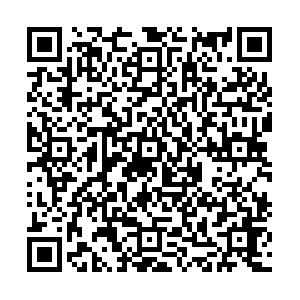# The effect of single-particle space-momentum angle distribution on two-pion HBT correlation in relativistic heavy-ion collisions using a multiphase transport model

• Using the string melting version of a multiphase transport (AMPT) model, we analyze the transverse momentum dependence of the HBT radius $R_{\rm s}$and the single-pion angle distribution on the transverse plane in central Au+Au collisions at $\sqrt{S_{NN}}$=19.6, 27, 39, 62.4, and 200 GeV. Additionally, a numerical connection between these two phenomena is established with a series of functions. We can estimate the single-pion angle distribution on the transverse plane from HBT analysis.
••Get Citation
Hang Yang, Qichun Feng, Yanyv Ren, Jingbo Zhang and Lei Huo. The effect of single-particle space-momentum angle distribution on two-pion HBT correlation in relativistic heavy-ion collisions by using a multiphase transport model[J]. Chinese Physics C. doi: 10.1088/1674-1137/ac1856
Hang Yang, Qichun Feng, Yanyv Ren, Jingbo Zhang and Lei Huo. The effect of single-particle space-momentum angle distribution on two-pion HBT correlation in relativistic heavy-ion collisions by using a multiphase transport model[J]. Chinese Physics C.Milestone
Article Metric

Article Views(92)
Cited by(0)
Policy on re-use
To reuse of subscription content published by CPC, the users need to request permission from CPC, unless the content was published under an Open Access license which automatically permits that type of reuse.
###### 通讯作者: 陈斌, bchen63@163.com
• 1.

沈阳化工大学材料科学与工程学院 沈阳 110142

Title:
Email:

## The effect of single-particle space-momentum angle distribution on two-pion HBT correlation in relativistic heavy-ion collisions using a multiphase transport model

###### Corresponding author: Jingbo Zhang, jinux@hit.edu.cn
• School of Physics, Harbin Institute of Technology, Harbin 150001, China

Abstract: Using the string melting version of a multiphase transport (AMPT) model, we analyze the transverse momentum dependence of the HBT radius $R_{\rm s}$and the single-pion angle distribution on the transverse plane in central Au+Au collisions at $\sqrt{S_{NN}}$=19.6, 27, 39, 62.4, and 200 GeV. Additionally, a numerical connection between these two phenomena is established with a series of functions. We can estimate the single-pion angle distribution on the transverse plane from HBT analysis.

### HTML关注分享DownLoad:  Full-Size Img  PowerPoint